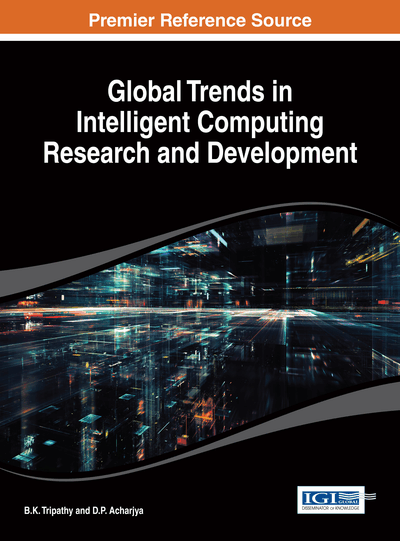# Learning Using Soft Computing Techniques

V. Susheela Devi (Indian Institute of Science, India)
DOI: 10.4018/978-1-4666-4936-1.ch003

## Abstract

This chapter focuses on a few key applications in the field of classification and clustering. Techniques of soft computing have been used to solve these applications. The first application finds a new similarity measure for time series data, combining some available similarity measures. The weight to be given to these similarity measures is found using a genetic algorithm. The other applications discussed are for pattern clustering. A Particle Swarm Optimization (PSO) algorithm has been used for clustering. A modification of the PSO using genetic operators has been suggested. In addition, simultaneous clustering and feature selection and simultaneous clustering and feature weighting has been discussed. Results have been given for all the techniques showing the improvement achieved using these techniques.
Chapter Preview
Top

## 1. Introduction

Soft Computing techniques generally refer to the techniques which use some method of search to solve the problem. It differs from conventional computing in that it is tolerant of imprecision, uncertainty, partial truth and approximation. It also refers to techniques which mimic the methods used by human beings and animals.

Some of the soft computing techniques are evolutionary computation, neural networks, particle swarm optimization, ant colony optimization, and fuzzy computing. This chapter talks about specific applications where the techniques mentioned above have been used. The applications are in the fields of supervised and unsupervised learning namely classification and clustering.

There are a number of classification methods like nearest neighbour, decision tree, support vector machines, rule based systems etc. It is also possible to use soft computing techniques like neural networks, evolutionary computations, particle swarm optimization etc. for carrying out classification. Even techniques for feature selection, feature extraction and prototype selection can be carried out using these techniques.

When the class labels of patterns are fuzzy, it means that each pattern belongs to each class with a membership value. When we use fuzzy classification techniques, we get the fuzzy membership value of each test pattern to every class which has to be defuzzified to get the crisp class label.

A rough set consists of two parts, the upper approximation which consists of objects which definitely belong to the set and the lower approximation which consists of objects which might belong to the set. In the context of pattern classification, each class is a rough set which has some patterns which belong to its upper approximation and some patterns which belong to its lower approximation. From the point of view of a pattern, it can belong to the upper approximation of one class or the lower approximation of more than one class.

Clustering can also be carried out using soft techniques like genetic algorithms, simulated annealing, particle swarm optimization etc.

The chapter will discuss the following topics:

• 1.

Use of genetic algorithms for time series classification (Dohare & Devi, 2011).

• 2.

Use of PSO for classification and clustering.

• 3.

Modified PSO with genetic operators for clustering(Swetha & Devi, 2012).

• 4.

Use of PSO for feature selection and clustering simultaneously(Swetha & Devi, 2012).

• 5.

Use of PSO for feature weighting and clustering simultaneously(Swetha & Devi, 2012)

Top

## 2. Genetic Algorithms For Time Series Classification

Distance-based time series classification can be done using a distance measure. There are a number of distance measures each of them have their own strengths and weaknesses. The distance measure used is crucial to the performance of the classification algorithm. The method discussed in this section, combines a number of distance measures by assigning weights to each one. The weights assigned are found by using a genetic algorithm.

Time series data consists of multivariate data where the values of one or more variable is in the form of a sequence. Time series data are found everywhere, for example, weather data, stocks and share prices, energy consumption at a place etc. These consist of data which vary in time. Generally, a time seriest = [t1, ..., tr],is an ordered set of r data points which are typically measured at successive points of time spaced at uniform time intervals. A time series could be a pattern which belongs to a particular class. The problem of time series classification is to learn a classifier C, which is a function that maps a time series t to a class label l, that is, C(t) = l where l ∈ L, the set of class labels.

There are three basic methods of carrying out time series classification:

## Complete Chapter List

Search this Book:
Reset